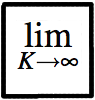# Non-parametric Bayesian Models

Non-parametric models are very flexible statistical models in which the complexity of the model grows with the amount of observed data. While traditional parametric models make strong assumptions about how the data was generated, non-parametric models try to make weaker assumptions and let the data "speak for itself". Many non-parametric models can be seen as infinite limits of finite parametric models, and an important family of non-parametric models are derived from Dirichlet processes.

See also Gaussian Processes.

Some relevant publications: